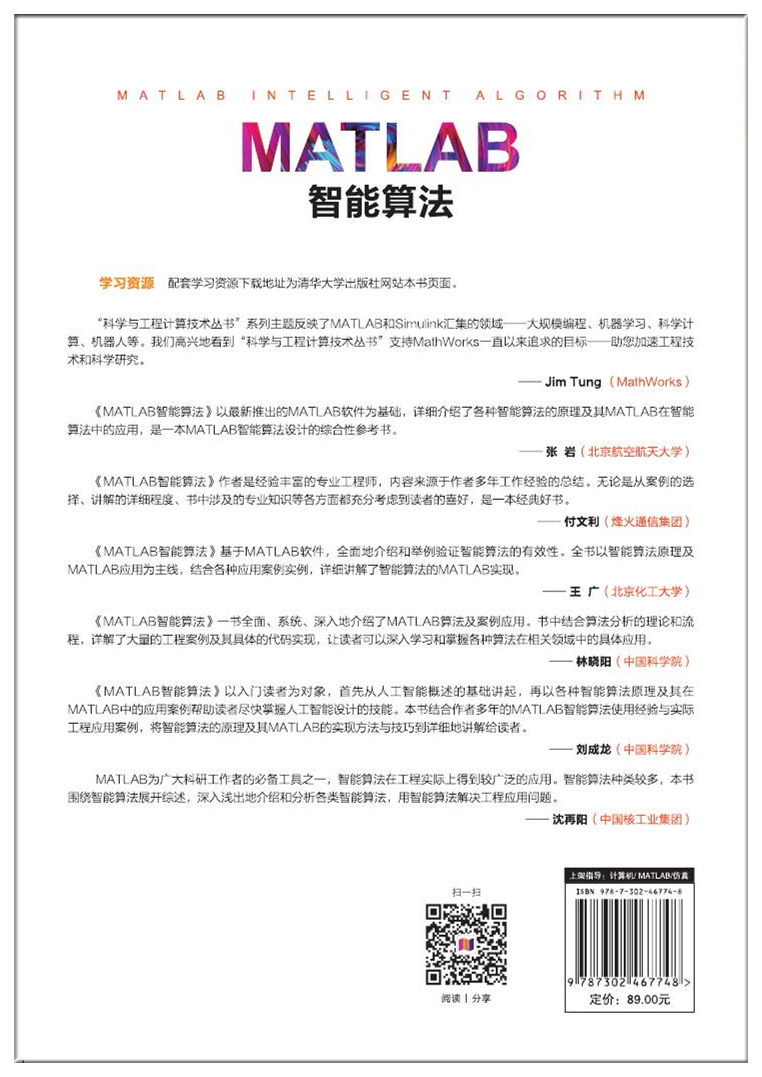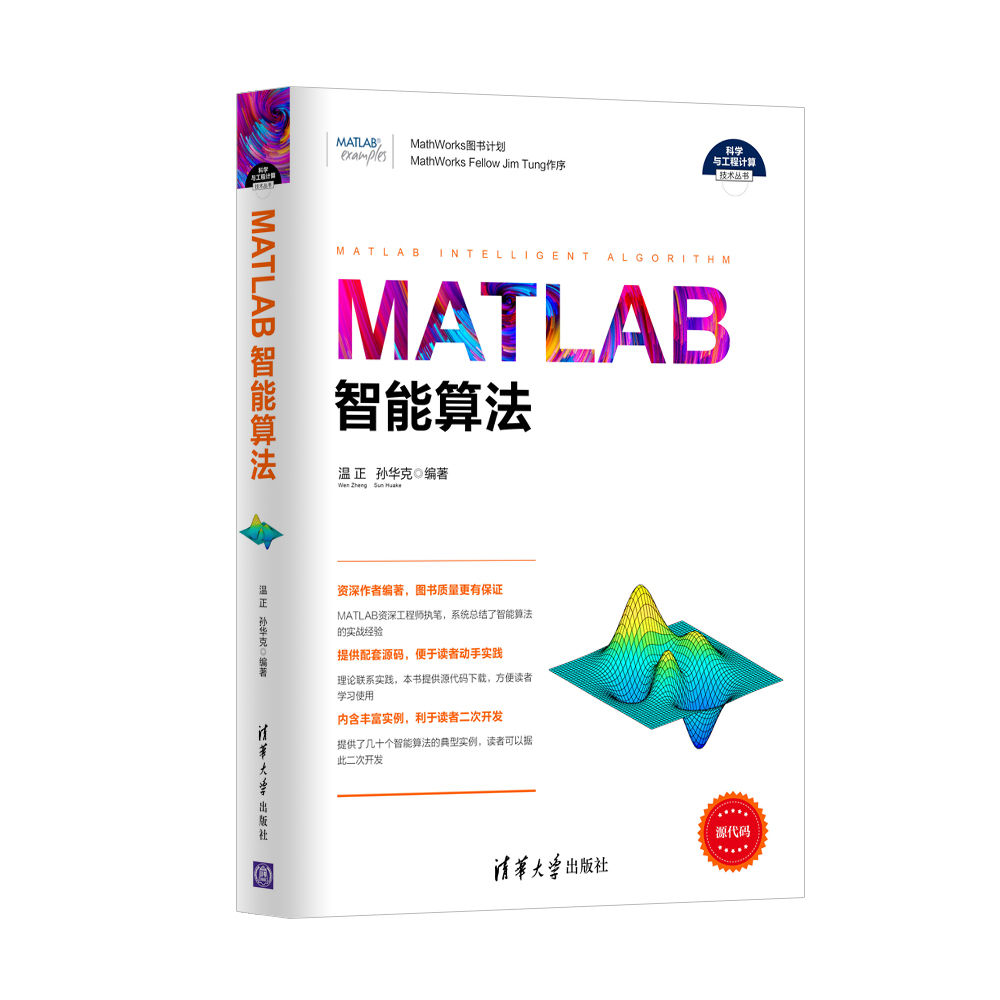## home > Book Center >

•••#### MATLAB-based Intelligence Algorithm

Author： Wen Zheng, Sun Huake
Impression：1-11
ISBN：9787302467748
Subject：Engineering
Publication Date：2017.09.01
Page Count：500

Based on the latest MATLAB 2016a, this book elaborates on the rationale of various intelligence algorithms and application of MATLAB in intelligence algorithms. It is a comprehensive reference book on MATLAB-based intelligence algorithms. This book encompasses 2 parts which are further divided into 13 chapters. Part 1 starts with an overview of AI, and then carefully introduces neural network algorithm, particle swarm optimization, genetic algorithm, fuzzy logic control, immune algorithm, ant colony algorithm, wavelet analysis algorithm, and MATLAB implementation. Part 2 introduces the applications of intelligence algorithms in engineering, including application of fuzzy neural network in engineering, application of genetic algorithm in image processing, application of neural networks in parameter estimation, PID control based on intelligence algorithm, and comprehensive application of intelligence algorithms.

more >

## Subjects

• Wen Zheng, holding a post-doctoral position in Beijing University of Aeronautics and Astronautics, is now working in an aerospace research institute. Wen is expert in engineering simulation and calculation software such as MATLAB, ANSYS and Fluent, and also writer of several best-selling computer-related books.

Copyright (C) 2014 Tsinghua University Press Ltd ICP No. 05029640 No. 11010802013248

Traffic：     Contact | lawyers | Link | Piracy Report